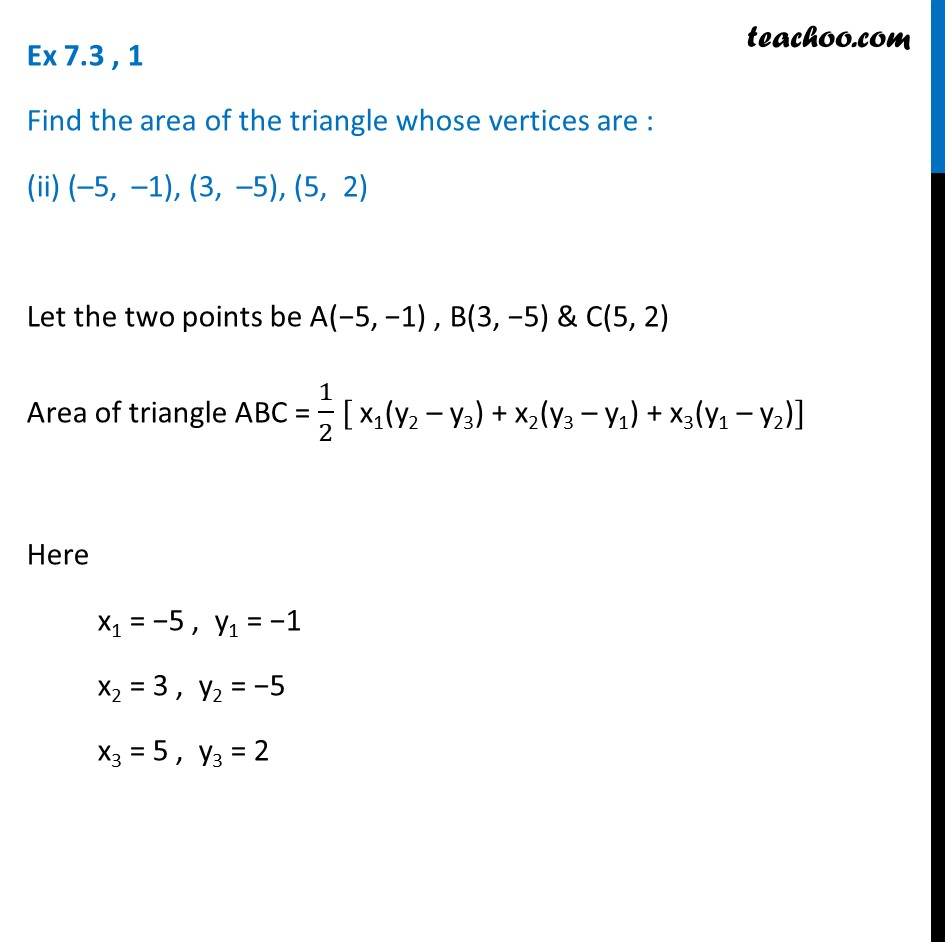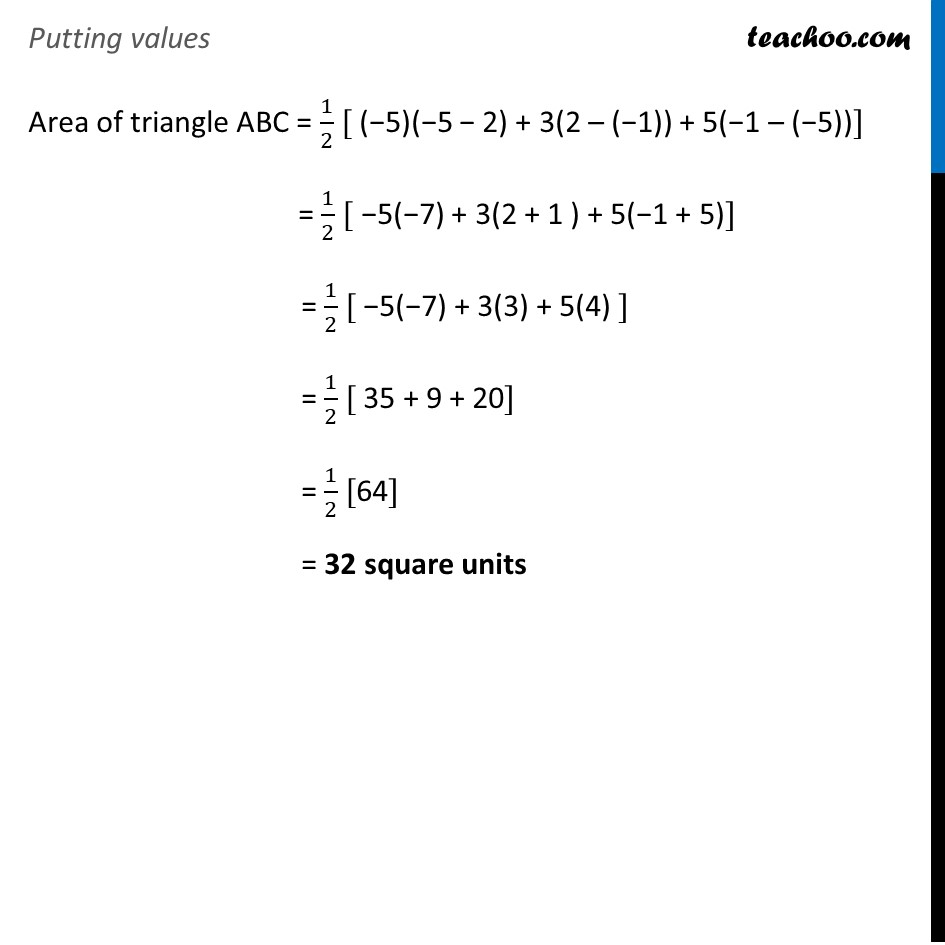Area of Triangle when coordinates are given

Chapter 7 Class 10 Coordinate Geometry
Serial order wiseLearn in your speed, with individual attention - Teachoo Maths 1-on-1 Class

### Transcript

Ex 7.3 , 1 Find the area of the triangle whose vertices are : (ii) (–5, –1), (3, –5), (5, 2) Let the two points be A(−5, −1) , B(3, −5) & C(5, 2) Area of triangle ABC = 1/2 [ x1(y2 – y3) + x2(y3 – y1) + x3(y1 – y2)] Here x1 = −5 , y1 = −1 x2 = 3 , y2 = −5 x3 = 5 , y3 = 2 Putting values Area of triangle ABC = 1/2 [ (−5)(−5 − 2) + 3(2 – (−1)) + 5(−1 – (−5))] = 1/2 [ −5(−7) + 3(2 + 1 ) + 5(−1 + 5)] = 1/2 [ −5(−7) + 3(3) + 5(4) ] = 1/2 [ 35 + 9 + 20] = 1/2  = 32 square units